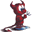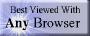music# Boolean logic

## summary

This subchapter looks at Boolean logic.

## free computer programming text book project

If you like the idea of this project,# postulates

## Boolean algebra

Boolean algebra is an algebraic system consisting of binary elements and binary operations.

The postulates of Boolean algebra provide the foundation for the entire system. The order of the postulates varies greatly from author to author. Some parts of postulates are not strictly necessary (might be derived as theorems instead), but in introductory materials such as this one, are filled out to make details clear to students.

Basic set: X, Y, and Z are elements of the set S.
Note that some authorities use the elements A, B, and C instead.

Equivalence: Equivalence is defined for the set S such that:
if X = Y and Y = Z
then X = Z

Operations: The operations + (Boolean addition) and · (Boolean multiplication) are defined such that:
X + Y and X · Y are in the set S
NOTE: These two operations were informally introduced in the introduction chapter.

Values: All elements in the set S will take on the valuation:
S = (0,1)
NOTE: These two values are often interpreted as false (0) and true (1).

Complement Law: Each member of the set S has an inverse (or compliment), such that when X = 0, then ¬X = 1
NOTE: The compliment is also indicated by a “tick” mark after the variable (X') and by placing a bar (or horizontal line) over a variable.
NOTE: The Complement Law produces the following important relationships:
X · ¬X = 0
X + ¬X = 1

Identity Law: The value 1 is the identity for Boolean multiplication and the value 0 is the identity for Boolean addition.
NOTE: The Identity Law produces the following important relationships:
X + 0 = X
X + 1 = 1

Cummulative Law: Boolean addition and Boolean multiplication are both cummulative:
X + Y = Y + X
X · Y = Y · X

Distributive Law: The Distributive Law in Boolean algebra highlights its different nature from normal linear algebra (this law is not true for normal algebra):
X · ( Y + Z ) = ( X · Y ) + ( X · Z )
X + ( Y · Z ) = ( X + Y ) · ( X + Z )

There are slight variations and alternate axiomatizations in presentations of Boolean algebra. The following are often included as axioms in some works. These can be proven from the above. If these axioms are used, then some of the above might become theorems.

Duality Principle: Duality holds that for any valid expression of identity, the resulting expression obtained by interchanging 1 and 0 and · and +, is a valid dual.
NOTE: This gives:
for the expression: X + ¬X = 1
the dual is: X · ¬X = 0

Idempotent Law: Property of a variable operating on itself.
X + X = X
X · X = X

Associative Law: Boolean addition and Boolean multiplciation are both associative.
X · ( Y · Z ) = ( X · Y ) · Z
X + ( Y + Z ) = ( X + Y ) + Z

Absorption Law:
X · ( X + Y ) = X
X + ( X · Y ) = X

Null Law:
X + 1 = 1
X · 0 = 0

Note that Boolean algebra does not have a subtraction or a division operation, but it does have a complement operation that isn’t found in normal linear algebra.

# free music player coding example

Coding example: I am making heavily documented and explained open source code for a method to play music for free — almost any song, no subscription fees, no download costs, no advertisements, all completely legal. This is done by building a front-end to YouTube (which checks the copyright permissions for you).

View music player in action: www.musicinpublic.com/.

Create your own copy from the original source code/ (presented for learning programming).

## view text bookHTML file

Because I no longer have the computer and software to make PDFs, the book is available as an HTML file, which you can convert into a PDF.

 Tweets by @osdata

## free computer programming text book project

Building a free downloadable text book on computer programming for university, college, community college, and high school classes in computer programming.

If you like the idea of this project,

send donations to:
Milo
PO Box 1361
Tustin, California 92781

Supporting the entire project:

If you have a business or organization that can support the entire cost of this project, please contact Pr Ntr Kmt (my church)

Some or all of the material on this web page appears in theThis web site handcrafted on Macintoshcomputers using Tom Bender’s Tex-Edit Plusand served using FreeBSD.†UNIX used as a generic term unless specifically used as a trademark (such as in the phrase “UNIX certified”). UNIX is a registered trademark in the United States and other countries, licensed exclusively through X/Open Company Ltd.

Names and logos of various OSs are trademarks of their respective owners.